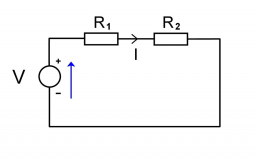# Resistances 66064

Two resistors with resistances of 40 ohms and 60 ohms are connected in series and connected to a voltage of 150V.
A) the total resistance
B) current in the circuit
C) voltage on both resistors

R =  100 Ω
I =  1.5 A
U1 =  60 V
U2 =  90 V

### Step-by-step explanation:Did you find an error or inaccuracy? Feel free to write us. Thank you!

Tips for related online calculators
Need help calculating sum, simplifying, or multiplying fractions? Try our fraction calculator.